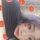# Коллоквиум. Automorphisms of Weyl Algebra

Лекция
Предмет:
Дата записи:
18.04.19
Дата публикации:
24.04.19
Код для блога:

The famous Jacobian Conjecture states that locally invertible polynomial mapping over is globally invertible. Dixmier conjecture says that any endomorphism of the ring of differential operators (Weyl algebra ) is an automorphism. In the paper A. Ya. Kanel-Belov and M. L. Kontsevich, «The Jacobian conjecture is stably equivalent to the Dixmier conjecture», Mosc. Math. J., 7:2 (2007), 209-218, arXiv:math/0512171, we constructed a homomorphism between the automorphism semigroup of and polynomial symplectomorphisms of Kontsevich Conjecture states that this homomorphism is an isomorphism of invertible mappings. In fact, Kontsevich’s Conjecture states that deformation quantization of affine space preserves the group of symplectic polynomial automorphisms, i.e. the group of polynomial symplectomorphisms in dimension 2n is canonically isomorphic to the group of automorphisms of the corresponding n-th Weyl algebra. The conjecture is confirmed for n=1 and open for n>1. We play with Plank constants and use singularity trick to confirm the general case of the conjecture, see Alexei Kanel-Belov, Andrey Elishev, Jie-Tai Yu, Augmented Polynomial Symplectomorphisms and Quantization, 2018, 21 pp., arXiv:1812.02859.

17

## КомментарииХорошо,мне нравится это видео.